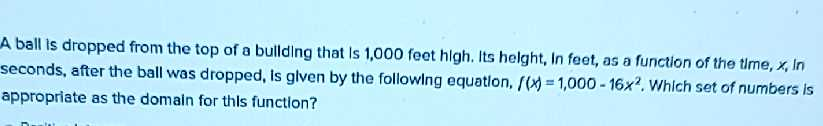### ¿Todavía tienes preguntas de matemáticas?

Pregunte a nuestros tutores expertos
Algebra
PreguntaA ball is dropped from the top of a bullding that Is $$1,000$$ feet high. Its helght, in feet, as a function of the time, $$x$$ , In seconds, after the ball was dropped, is glven by the following equation, $$f ( x ) = 1,000 - 16 x ^ { 2 }$$ . Which set of numbers is appropriate as the domain for this function?